# direnum.c File Reference

Directory enumerator functions. More...

`#include <fcntl.h>`
`#include <sys/stat.h>`
`#include <sys/types.h>`
`#include <errno.h>`
`#include <unistd.h>`
`#include <stdlib.h>`
`#include <stdio.h>`
`#include <string.h>`
`#include "est_ops.h"`
`#include "direnum.h"`
`#include "warnings.h"`
`#include "global.h"`
`#include "helper.h"`
`#include <linux/types.h>`
`#include <linux/unistd.h>`
Include dependency graph for direnum.c: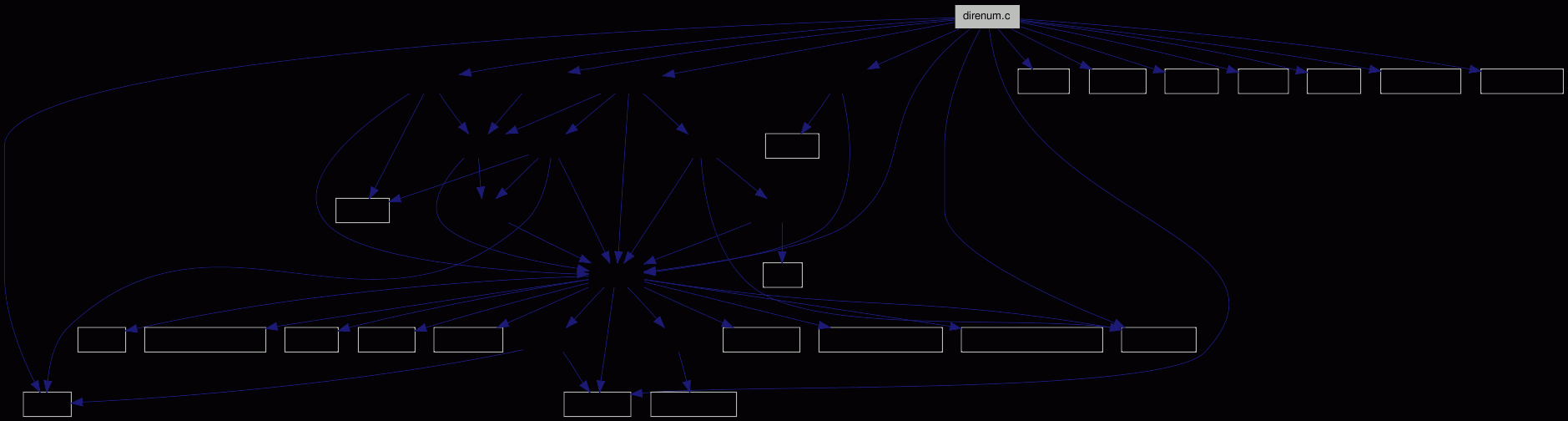Go to the source code of this file.

## Defines

#define HAVE_GETDENTS64   1
If the system fulfills all necessary checks to use getdents(), this macro is set.
#define FREE_SPACE   (4096)
The amount of memory that should be allocated for directory reading.

## Typedefs

typedef int dir__handle
The type of handle.
typedef struct dirent64 fsvs_dirent
A compatibility structure.

## Functions

int dir__start_enum (dir__handle *dh, char *path)
Starts enumeration of the given path.
int dir__enum (dir__handle dh, fsvs_dirent *dirp, unsigned int count)
The enumeration function.
int dir__close (dir__handle dh)
Simply closes the handle dh.
int dir__get_dir_size (dir__handle dh, struct sstat_t *st)
How to get the length of a directory (in bytes), from a handle dh, into st->size.
int dir___f_sort_by_inodePP (struct estat *a, struct estat *b)
Compares two struct estat pointers by device/inode.
int dir___f_sort_by_inode (struct estat **a, struct estat **b)
Compares the data inside two struct estat pointers to pointers by device/inode.
int dir___f_sort_by_nameCC (const void *a, const void *b)
Compares two names/strings.
int dir___f_sort_by_name (const void *a, const void *b)
Compares the data inside two struct estat pointers to pointers by name.
int dir___f_sort_by_nameCS (const void *a, const void *b)
Compares a pointer to name (string) with a struct estat pointer to pointer.
int dir__sortbyname (struct estat *sts)
-.
int dir__sortbyinode (struct estat *sts)
-.
int dir__enumerator (struct estat *this, int est_count, int give_by_name)
-.

## Detailed Description

Directory enumerator functions.

Definition in file direnum.c.

## Define Documentation

 #define FREE_SPACE   (4096)

The amount of memory that should be allocated for directory reading.

This value should be bigger (or at least equal) than the number of bytes returned by getdents(). For the compatibility layer it's more or less the maximum filename length plus the inode and record length lengths.

This many bytes more will also be allocated for the filenames in a directory; if we get this close to the end of the buffer, the memory area will be reallocated.

Definition at line 226 of file direnum.c.

Referenced by dir__enumerator().

## Function Documentation

 int dir___f_sort_by_inode ( struct estat ** a, struct estat ** b )

Compares the data inside two struct estat pointers to pointers by device/inode.

Returns:
+2, +1, 0, -1, -2, suitable for qsort().

Definition at line 252 of file direnum.c.

References dir___f_sort_by_inodePP().

Referenced by dir__sortbyinode(), ops__find_entry_byinode(), waa___find_position(), and waa__output_tree().

Here is the call graph for this function: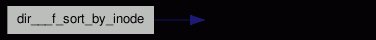Here is the caller graph for this function:int dir___f_sort_by_inodePP ( struct estat * a, struct estat * b )

Compares two struct estat pointers by device/inode.

Returns:
+2, +1, 0, -1, -2, suitable for qsort().

That is now an inline function; but without force gcc doesn't inline it on 32bit, because of the size (64bit compares, 0x6b bytes). [ `__attribute__`((always_inline)) in declaration].

Definition at line 235 of file direnum.c.

References sstat_t::dev, sstat_t::ino, and estat::st.

Referenced by dir___f_sort_by_inode(), and ign__is_ignore().

Here is the caller graph for this function: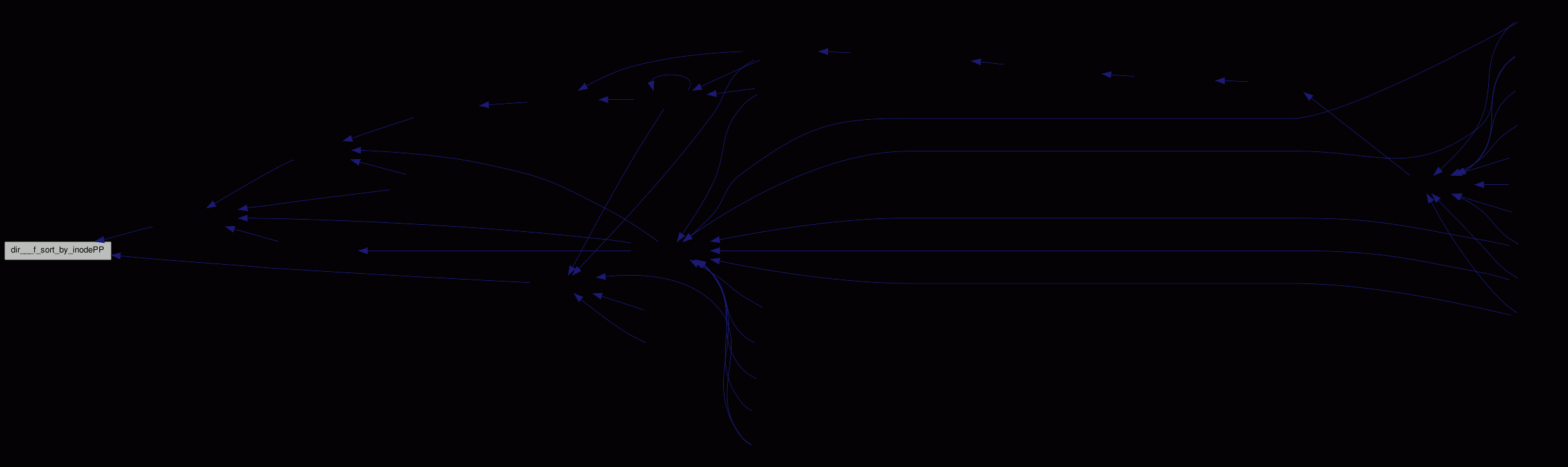int dir___f_sort_by_name ( const void * a, const void * b )

Compares the data inside two struct estat pointers to pointers by name.

Returns:
+2, +1, 0, -1, -2, suitable for qsort().

Definition at line 271 of file direnum.c.

References dir___f_sort_by_nameCC().

Referenced by dir__sortbyname(), and ops__correlate_dirs().

Here is the call graph for this function: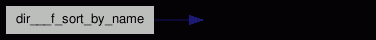Here is the caller graph for this function:int dir___f_sort_by_nameCC ( const void * a, const void * b ) ` [inline]`

Compares two names/strings.

Used for type checking cleanliness. 'C' as for 'Const'.

Returns:
+2, +1, 0, -1, -2, suitable for qsort().

Definition at line 262 of file direnum.c.

Referenced by dir___f_sort_by_name(), and dir___f_sort_by_nameCS().

Here is the caller graph for this function:int dir___f_sort_by_nameCS ( const void * a, const void * b )

Compares a pointer to name (string) with a struct estat pointer to pointer.

Returns:
+2, +1, 0, -1, -2, suitable for qsort().

Definition at line 283 of file direnum.c.

References dir___f_sort_by_nameCC().

Referenced by ops__find_entry_byname().

Here is the call graph for this function: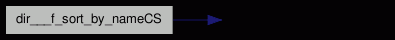Here is the caller graph for this function:int dir__enumerator ( struct estat * this, int est_count, int give_by_name )

-.

This function reads a directory into a self-allocated memory area.

The entries are sorted by inode number and stat()ed.

Parameters:
 this a pointer to this directory's stat - for estimating the number of entries. Only this->st.st_size is used for that - it may have to be zeroed before calling. est_count is used to give an approximate number of entries, to avoid many realloc()s. give_by_name simply tells whether the ->by_name array should be created, too.

The result is written back into the sub-entry array in this.

To avoid reallocating (and copying!) large amounts of memory, this function fills some arrays from the directory, then allocates the needed space, sorts the data (see note below) and adds all other data. See sts_array, names and inode_numbers.

Note:
Sorting by inode number brings about 30% faster lookup times on my test environment (8 to 5 seconds) on an empty cache. Once the cache is filled, it won't make a difference.
Returns:
0 for success, else an errorcode.

Definition at line 361 of file direnum.c.

Referenced by waa__dir_enum().

Here is the call graph for this function:Here is the caller graph for this function:int dir__sortbyinode ( struct estat * sts )

-.

Sorts the existing estat::by_inode array afresh, by device/inode.

Definition at line 322 of file direnum.c.

References BUG_ON, estat::by_inode, dir___f_sort_by_inode(), and estat::entry_count.

Referenced by dir__enumerator(), ops__find_entry_byinode(), and waa__output_tree().

Here is the call graph for this function: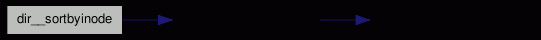Here is the caller graph for this function:int dir__sortbyname ( struct estat * sts )

-.

Sorts the entries of the directory sts by name into the estat::by_name array, which is reallocated and NULL-terminated.

If it has no entries, an array with NULL is nonetheless allocated.

Definition at line 293 of file direnum.c.

Referenced by dir__enumerator(), ops__correlate_dirs(), ops__find_entry_byname(), and waa__do_sorted_tree().

Here is the call graph for this function:Here is the caller graph for this function:Generated for fsvs by1.6.1